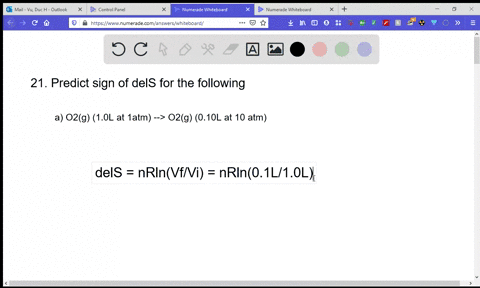Enroll in one of our FREE online STEM summer camps. Space is limited so join now!View Summer Courses### Predict the sign of $\Delta S$ for each process: …

07:37North Carolina State University

Need more help? Fill out this quick form to get professional live tutoring.

Get live tutoring
Problem 20

Predict the sign of $\Delta S$ for each process
$$\begin{array}{l}{\text { (a) } \mathrm{C}_{2} \mathrm{H}_{5} \mathrm{OH}(g)(350 \mathrm{K} \text { and } 500 \text { torr) } \longrightarrow} \\ {\mathrm{C}_{2} \mathrm{H}_{5} \mathrm{OH}(g)(350 \mathrm{K} \text { and } 250 \text { torr) }}\end{array}$$
$$\begin{array}{l}{\text { (b) } \mathrm{N}_{2}(g)(298 \mathrm{K} \text { and } 1 \mathrm{atm}) \longrightarrow \mathrm{N}_{2}(a q)(298 \mathrm{K} \text { and } 1 \mathrm{atm})} \\ {\text { (c) } \mathrm{O}_{2}(a q)(303 \mathrm{K} \text { and } 1 \mathrm{atm}) \longrightarrow \mathrm{O}_{2}(g)(303 \mathrm{K} \text { and } 1 \mathrm{atm})}\end{array}$$

Check back soon!

## Discussion

You must be signed in to discuss.